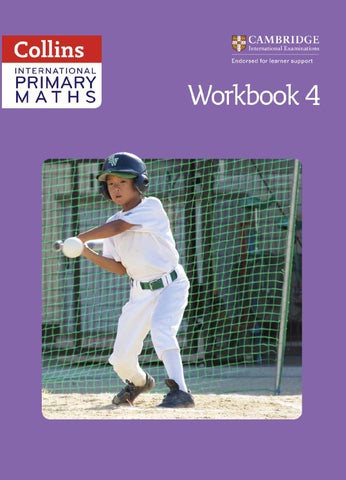6359_CIE_Maths_WB4_BOOK.indb i

04/08/2016 13:08

Contents Number 1 2 3 4 5 6 7 8 9 10 11 12

Whole numbers 1 Whole numbers 2 Whole numbers 3 Decimals 1 Decimals 2 Fractions Addition and subtraction 1 Addition and subtraction 2 Addition and subtraction 3 Multiplication and division 1 Multiplication and division 2 Multiplication and division 3

2 10 18 26 34 42 58 74 82 98 114 130

Geometry 13 2D shape, including symmetry 14 3D shape 15 Position and movement

146 162 170

Measure 16 17 18 19 20 21

Length Mass Capacity Measures Time Area and perimeter

178 186 194 202 210 218

Handling data 22 Handling data 1 23 Handling data 2

6359_CIE_Maths_WB4_BOOK.indb iii

226 234

04/08/2016 13:08

Unit

1

Whole numbers 1

Number

Lesson 1: Counting, reading and writing numbers â&#x20AC;˘ Read and write numbers to 10 000

â&#x20AC;˘ Recognise multiples of 5, 10 and 100 Challenge

1

1 Write these numbers using numerals. a one hundred and twenty-five b seven hundred and fifty-one c nine hundred and thirty-three d three hundred and nineteen e two hundred and forty-seven 2 Count on in 1s from each of these numbers. a 7921 7922 b 3567 3568 c 2412 2413 d 1974 1975

Challenge

2

1 a Draw a line to match each number in words with the same number written in numerals. 9465

six thousand four hundred and fifty-nine

6900

nine thousand four hundred and fifty

6459

six thousand nine hundred

9450

nine thousand four hundred and sixty-five

2

6359_CIE_Maths_WB4_BOOK.indb 2

04/08/2016 13:08

Student's Book page 1

Measure Number

b Which number in Question a is a multiple of 100? c Which is not a multiple of 5? 2 Write each distance in words. a Tokyo: 4382 km b Dubai: 2690 km c London: 1462 km d Madrid: 5039 km 3 a Fill in each gap as you count forwards and backwards in 1000s. 7597

1597 2597 9305 8305

b Fill in each gap as you count forwards and backwards in 100s. 2590 2690 1729 1629

3090 1429

Challenge

3 Use the digit cards to make the numbers. Write each number in numerals and words. 5 0 2 8 0 a A multiple of 100.

b An odd number.

c A multiple of 10.

3

6359_CIE_Maths_WB4_BOOK.indb 3

04/08/2016 13:08

1

Unit

Whole numbers 1

Lesson 2: Place value Number

â&#x20AC;˘ Know the value of each digit in a 4-digit number Challenge

1 Write the place value of each digit. a b

Challenge

2

c

d

e

f

1 Each of these forks shows a 4-digit number. Write the number under each fork and write the letter of the statement that fits. A The largest number. B A multiple of 100. C A number where the 4 digit is worth four 1000s. D A number with an odd number of 10s.

4

6359_CIE_Maths_WB4_BOOK.indb 4

04/08/2016 13:08

Studentâ&#x20AC;&#x2122;s Book page 2

Example:

3592

=

3000

+

500

+

a 2634 =

+

+

+

b 5217 =

+

+

+

90

+

Number

2 Nia makes these 4-digit numbers using corn on a fork. Write down how each number can be partitioned. 2

c eight thousand one hundred and sixty-two =

+

+

+

Challenge

3 You have only eight beads.

a What is the largest 4-digit number you can make?

b What is the smallest 4-digit number you can make?

c Look carefully at this list. Write another eight numbers that only use eight beads and begin with a 5 in the 1000s place. 8000

7100

7010

7001

6200

6020

6002

6110

6101

6011

5

6359_CIE_Maths_WB4_BOOK.indb 5

04/08/2016 13:08

Unit

1

Whole numbers 1

Lesson 3: Rounding whole numbers Number

â&#x20AC;˘ Round 3- and 4-digit numbers

You will need â&#x20AC;˘ coloured pencils

to the nearest 10 or 100

Challenge

1

1 Colour each of the numbers that rounds to 840.

841

848

837

839

845

832

2 Colour each of the numbers that rounds to 920.

928

921

915

919

922

929

3 Colour each of the numbers that rounds to 300 when rounded to the nearest 100.

314

385

250

234

393

276

4 Colour each of the numbers that rounds to 600 when rounded to the nearest 100.

638 Challenge

2

684

549

579

620

511

1 Mark each number on the number line and show whether you would round it up or down to the nearest 10. a 3782 3780

3790

4290

4300

6510

6520

1020

1030

b 4297

c 6514

d 1025 6

6359_CIE_Maths_WB4_BOOK.indb 6

04/08/2016 13:08

2 Mark each number on the number line and show whether you would round it up or down to the nearest 100. a 5294

b 1852

c 4407

d 2650

e 7290

5200

5300

1800

1900

4400

4500

2600

2700

7200

7300

Number

Studentâ&#x20AC;&#x2122;s Book page 3

Challenge

3

If I add the digits of my mystery 4-digit number, they make 25. If I round my mystery number to the nearest hundred, it rounds to 9000. What could Rajeshâ&#x20AC;&#x2122;s number be?

Rajesh

Invent a similar puzzle of your own for a friend to try.

7

6359_CIE_Maths_WB4_BOOK.indb 7

04/08/2016 13:08